## 0x2：解决条件概率预测的两个学术分支

SVM（支持向量机模型）：

SVM的决策面只和训练集中的少数实例有关，不会因为某些实例的变化而改变分界面，这从理论上证明了过拟合的发生
SVM的决策面考虑了最大化原理类间实例距离，这提供了更强的泛化能力，缓解了欠拟合的发生

……## 0x3：什幺场景适合贝叶斯网建模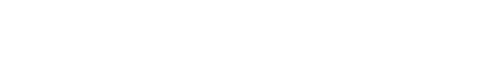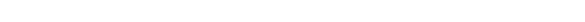`《数据挖掘 实用机器学习工具与技术》Ian H.Witten Eibe Frank，Mark A.Hall`

## 0x1：一个关于天气预测的贝叶斯网预测示例play类属性：先验概率分别为0.633和9.367，分别和4个属性结点存在一条有向边， 实际上，这个贝叶斯网退化成了一个朴素贝叶斯结构
outlook属性：在先验play的条件下，分别有：sunny、overcast、rainy这3个后验概率属性
windy属性：在先验play的条件下，分别有：false、true这2个后验概率属性
temperature属性：在先验play的条件下，分别有：hot、mild、cool这3个后验概率属性
humidity属性：在先验play的条件下，分别有：high、normal这2个后验概率属性

## 0x2：一个稍微复杂一些的天气预测例子### 1. 基于贝叶斯概率链式法则计算实例每个类值概率

P（play=no）= 0.367
P（outlook=rainy | play=no）= 0.385
P（temperature=cool | play=no，outlook=rainy）= 0.429
P（humidity=high | play=no，temperature=cool）= 0.250
P（windy=true | play=no，outlook=rainy）= 0.167

P（play=no，outlook=rainy，temperature=cool，humidity=high，windy=true）=

P（play=no）*
P（windy=true | play=no，outlook=rainy）
P（outlook=rainy | play=no）*
P（temperature=cool | play=no，outlook=rainy）*
P（humidity=high | play=no，temperature=cool）*
= 0.367 * 0.385 * 0.429 * 0.250 * 0.167 = 0.00253069942125

#### 【play=？ outlook=overcast ，temperature=cool，humidity=high，windy=true】

outlook属性值的不同，使概率链条进入了另一个分布空间

### 2. 贝叶斯网概率计算中链式分解法则的解释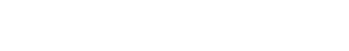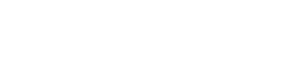P(play=?，windy=?，outlook=?，humidity=?，temperature=?) =

P(play)
P(windy | play)
P(outlook | windy，play) *
P(temperature | outlook，windy，play) *
P(humidity | temperature，toutlook，windy，play) *

P(play=?，windy=?，outlook=?，humidity=?，temperature=?) =

P(play) = P(play)
P(windy | play) = P(windy | play，outlook)
P(outlook | windy，play) = P(outlook | play)
P(temperature | toutlook，windy，play) = P(temperature | outlook，play)
P(humidity | temperature，toutlook，windy，play) = P(humidity | temperature，play)

P（play=no，outlook=rainy，temperature=cool，humidity=high，windy=true）=

P（play=no）*
P（windy=true | play=no，outlook=rainy）
P（outlook=rainy | play=no）*
P（temperature=cool | play=no，outlook=rainy）*
P（humidity=high | play=no，temperature=cool）*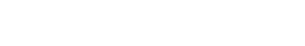a 1 , a 2 ,.. 代表图中每个节点， P a r ( a i) 就表示除了a i 以外的所有节点。公式中的逗号表示“与”的关系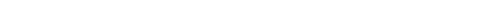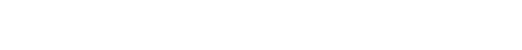## 0x3：警惕因果推断陷阱

`https://www.cnblogs.com/LittleHann/p/11683607.html#_label3_2_1_1`

## 4. 贝叶斯网训练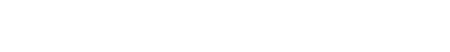## 0x1：贝叶斯网结点结构学习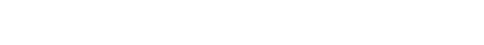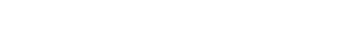“网络结构质量评估方法” ：基于局部条件独立性进行概率累计运算
“网络拓朴空间搜索方法” ：如何在指数级的穷举空间中有效地进行拓朴搜索

### 1. 基于某个数据集的网络结构质量评估函数

Akaike信息准则（Akaike Information Criterion，AIC）：AIC得分 = -LL + K

K为参数数量

### 2. 网络结点有向边连接空间搜索策略

#### 1）K2算法

K2算法是一个简单而快速的启发式算法，简要描述如下：

#### 5）HHMC启发式搜索算法

`https://www.google.com.hk/search?newwindow=1&safe=strict&hl=zh-CN&sxsrf=ACYBGNQ6fvIClIfRwRyXUasIvDRpeZpjEQ%3A1574044570225&source=hp&ei=mgPSXYb0Cs6AtgX18K6QBg&q=mmhc.r+&oq=mmhc.r+&gs_l=psy-ab.3...1738.1738..1925...0.0..0.0.0.......0....2j1..gws-wiz.&ved=0ahUKEwiGqd373PLlAhVOgK0KHXW4C2IQ4dUDCAY&uact=5`

## 0x2：贝叶斯网结点条件概率学习`《数据挖掘 实用机器学习工具与技术》Ian H.Witten Eibe Frank，Mark A.Hall`

## 0x1：贝叶斯网最大后验概率估计推断## 0x1：基本使用介绍

### 1. 从已知模型参数中创建概率分布

```# -*- coding:utf-8 -*-
from pomegranate import *
import pygraphviz as pgv
import matplotlib.pyplot as plt

def plot_xy(x, y):
plt.plot(x, y)
plt.show()

if __name__ == '__main__':
mu, sig = 0, 2
model = NormalDistribution(mu, sig)
x_y = {}
for i in range(9999):
x = float("%.1f" % model.sample())
if x_y.has_key(x):
x_y[x] += 1
else:
x_y[x] = 1
x_y = sorted(x_y.items(), key=lambda d: d)
print x_y
x = [i for i in x_y]
y = [i for i in x_y]
print x
print y
plot_xy(x, y)```### 2. 从数据中直接学习概率分布

```# -*- coding:utf-8 -*-
from pomegranate import *
import pygraphviz as pgv
import matplotlib.pyplot as plt
import numpy as np

def plot_xy(x, y):
plt.plot(x, y)
plt.show()

def generate_data():
X = np.random.normal(0, 1, 100)
model = NormalDistribution.from_samples(X)
return model

def generate_xy_plot(model):
x_y = {}
for i in range(9999):
x = float("%.1f" % model.sample())
if x_y.has_key(x):
x_y[x] += 1
else:
x_y[x] = 1
x_y = sorted(x_y.items(), key=lambda d: d)
print x_y
x = [i for i in x_y]
y = [i for i in x_y]
print x
print y
return x,y

if __name__ == '__main__':
model = generate_data()
x, y = generate_xy_plot(model)
plot_xy(x, y)```### 3. HMM detection```# -*- coding:utf-8 -*-
from pomegranate import *
import pygraphviz as pgv
import matplotlib.pyplot as plt
import numpy as np

if __name__ == '__main__':
d1 = DiscreteDistribution({
'A': 0.25,
'C': 0.25,
'G': 0.25,
'T': 0.25
})
d2 = DiscreteDistribution({
'A': 0.10,
'C': 0.40,
'G': 0.40,
'T': 0.10
})
s1 = State(d1, name="background")   # 隐状态
s2 = State(d2, name="CG island")    # 显状态
hmm = HiddenMarkovModel("CG-detector")
# 状态概率转移矩阵
# 隐状态和显状态的概率发射矩阵
hmm.bake()
#
observed_sequence = "GACTACGACTCGCGCTCGCGCGACGCGCTCGACATCATCGACACGACACTC"
print "how likely the sequence happen: ", hmm.log_probability(list(observed_sequence))
print "the corresponding hidden state sequence: ", hmm.predict(list(observed_sequence))```### 4. 贝叶斯网建模”Monty Hall problem“

```# -*- coding:utf-8 -*-
from pomegranate import *
import pygraphviz as pgv
import matplotlib.pyplot as plt
import numpy as np

if __name__ == '__main__':
guest = DiscreteDistribution({'A': 1. / 3, 'B': 1. / 3, 'C': 1. / 3})
prize = DiscreteDistribution({'A': 1. / 3, 'B': 1. / 3, 'C': 1. / 3})
monty = ConditionalProbabilityTable(
[['A', 'A', 'A', 0.0],
['A', 'A', 'B', 0.5],
['A', 'A', 'C', 0.5],
['A', 'B', 'A', 0.0],
['A', 'B', 'B', 0.0],
['A', 'B', 'C', 1.0],
['A', 'C', 'A', 0.0],
['A', 'C', 'B', 1.0],
['A', 'C', 'C', 0.0],
['B', 'A', 'A', 0.0],
['B', 'A', 'B', 0.0],
['B', 'A', 'C', 1.0],
['B', 'B', 'A', 0.5],
['B', 'B', 'B', 0.0],
['B', 'B', 'C', 0.5],
['B', 'C', 'A', 1.0],
['B', 'C', 'B', 0.0],
['B', 'C', 'C', 0.0],
['C', 'A', 'A', 0.0],
['C', 'A', 'B', 1.0],
['C', 'A', 'C', 0.0],
['C', 'B', 'A', 1.0],
['C', 'B', 'B', 0.0],
['C', 'B', 'C', 0.0],
['C', 'C', 'A', 0.5],
['C', 'C', 'B', 0.5],
['C', 'C', 'C', 0.0]], [guest, prize])
s1 = Node(guest, name="guest")
s2 = Node(prize, name="prize")
s3 = Node(monty, name="monty")
model = BayesianNetwork("Monty Hall Problem")
model.bake()
model.plot()```

### 5. 贝叶斯网问题预测Guest选择门编号的条件概率

```# -*- coding:utf-8 -*-
from pomegranate import *
import pygraphviz as pgv
import matplotlib.pyplot as plt
import numpy as np

if __name__ == '__main__':
guest = DiscreteDistribution({'A': 1. / 3, 'B': 1. / 3, 'C': 1. / 3})
prize = DiscreteDistribution({'A': 1. / 3, 'B': 1. / 3, 'C': 1. / 3})
monty = ConditionalProbabilityTable(
[['A', 'A', 'A', 0.0],
['A', 'A', 'B', 0.5],
['A', 'A', 'C', 0.5],
['A', 'B', 'A', 0.0],
['A', 'B', 'B', 0.0],
['A', 'B', 'C', 1.0],
['A', 'C', 'A', 0.0],
['A', 'C', 'B', 1.0],
['A', 'C', 'C', 0.0],
['B', 'A', 'A', 0.0],
['B', 'A', 'B', 0.0],
['B', 'A', 'C', 1.0],
['B', 'B', 'A', 0.5],
['B', 'B', 'B', 0.0],
['B', 'B', 'C', 0.5],
['B', 'C', 'A', 1.0],
['B', 'C', 'B', 0.0],
['B', 'C', 'C', 0.0],
['C', 'A', 'A', 0.0],
['C', 'A', 'B', 1.0],
['C', 'A', 'C', 0.0],
['C', 'B', 'A', 1.0],
['C', 'B', 'B', 0.0],
['C', 'B', 'C', 0.0],
['C', 'C', 'A', 0.5],
['C', 'C', 'B', 0.5],
['C', 'C', 'C', 0.0]], [guest, prize])
s1 = Node(guest, name="guest")
s2 = Node(prize, name="prize")
s3 = Node(monty, name="monty")
model = BayesianNetwork("Monty Hall Problem")
model.bake()
print model.probability([['A', 'A', 'A'],
['A', 'A', 'B'],
['C', 'C', 'B']])``````https://www.ctolib.com/jmschrei-pomegranate.html
https://blog.laisky.com/p/pygraphviz/
http://www.sohu.com/a/150274125_505915
https://github.com/jmschrei/pomegranate/blob/master/docs/BayesianNetwork.rst

## 7. bnlearn

```http://www.bnlearn.com/examples/